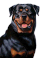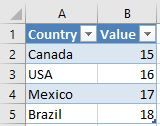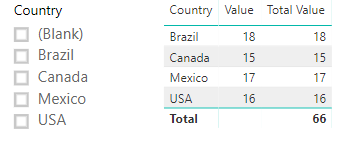cancel
Showing results for
Did you mean:Helper I

## Specific filter doubt

Hello everyone!

Any help here is appreciated! Considering the following table example:The current report is running the VALUE column with a standard SUM formula and is filtered by the COUNTRY column with a filter visualization.

I'd like to know if it's possible (and how to) to add on the formula the following context: if the filter equals "Brasil" then add 100.

Diego

1 ACCEPTED SOLUTIONMicrosoft

Hi @Tulio_DL,

```Total Value =
IF (
CALCULATE ( ISFILTERED ( Table1[Country] ), ALLSELECTED ( Table1 ) ),
IF (
SELECTEDVALUE ( Table1[Country] ) = "Brazil",
SUM ( Table1[Value] ) + 100,
SUM ( Table1[Value] )
),
SUM ( Table1[Value] )
)```Best regards,

Yuliana Gu

Community Support Team _ Yuliana Gu
If this post helps, then please consider Accept it as the solution to help the other members find it more quickly.
4 REPLIES 4Microsoft

Hi @Tulio_DL,

```Total Value =
IF (
CALCULATE ( ISFILTERED ( Table1[Country] ), ALLSELECTED ( Table1 ) ),
IF (
SELECTEDVALUE ( Table1[Country] ) = "Brazil",
SUM ( Table1[Value] ) + 100,
SUM ( Table1[Value] )
),
SUM ( Table1[Value] )
)```Best regards,

Yuliana Gu

Community Support Team _ Yuliana Gu
If this post helps, then please consider Accept it as the solution to help the other members find it more quickly.Helper I

Thank you very much @v-yulgu-msftHelper I
Create new column instead of mesure and use this formula ->If( [Country]="Brazil", [Value]+100,[value])Helper I

Hello Shantanu!

Thank you for participating man, what you've said is a way to do the trick.... But I still need to know if there's a way to do what I've pointed out on the original post because my data table is a lot more complex than this example.